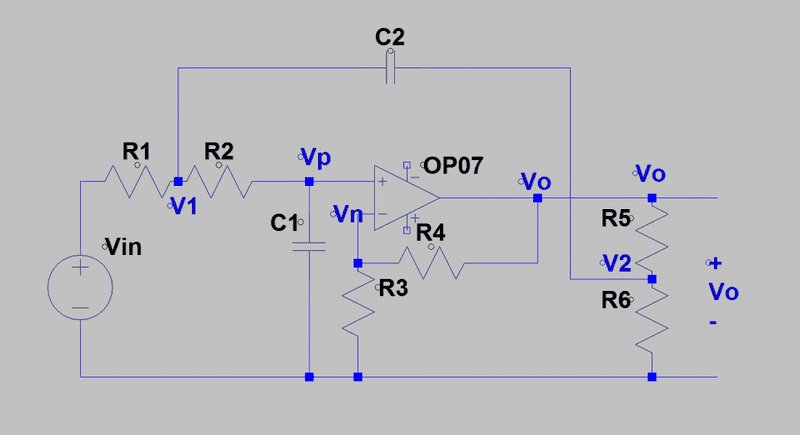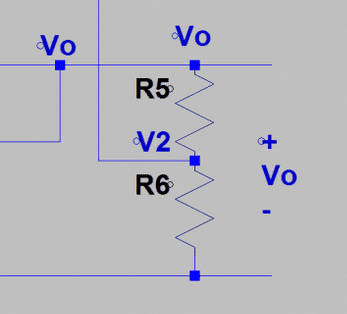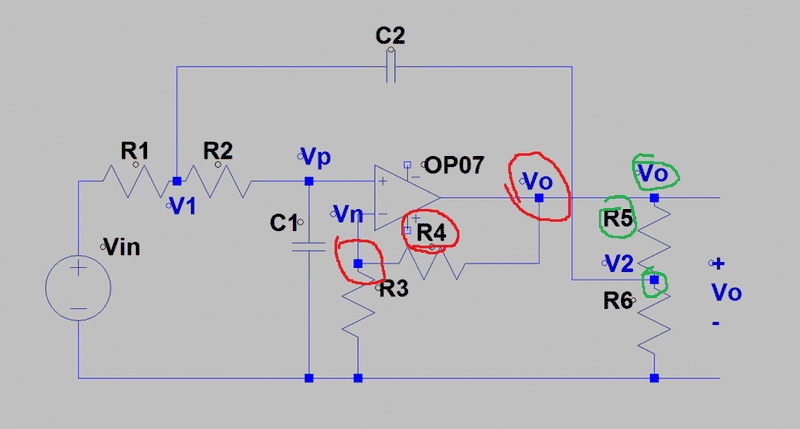# OpAmp circuit analysis

• Engineering

## Homework StatementFind the differential equation for Vo

KCL

## The Attempt at a Solution

At node v1:
##\frac{V_1-V_{in}}{R_1}+\frac{V_1-V_p}{R_2}+C_2(\dot{V_1}-\dot{V_2})=0##
At node vp:
##C_1\dot{V_P}+\frac{V_P-V_1}{R_2}=0##
At node vn:
##\frac{V_N}{R_3}+\frac{V_N-V_o}{R_4}=0##
At node v2:
##\frac{V_2}{R_6}+\frac{V_2-V_o}{R_5}+C_2(\dot{V_2}-\dot{V_1})=0##
At node Vo:
##\frac{V_o-V_N}{R_4}+\frac{V_o-V_2}{R_5}=0##

This is my attempt at solving for Vo:
Take equation of node VP and solve for V1
##C_1\dot{V_P}+\frac{V_P-V_1}{R_2}=0##
##V_1=R_2C_1\dot{V_P}+V_P##
derivative
##\dot{V_1}=R_2C_1\ddot{V_P}+\dot{V_P}##

Take equation of node Vo and solve for V2
##\frac{V_o-V_N}{R_4}+\frac{V_o-V_2}{R_5}=0##
##V_2 = \frac{R_5}{R_4}(V_o-V_{N})+V_o##
derivative
##\dot{V_2} = \frac{R_5}{R_4}(\dot{V_o}-\dot{V_{N}})+\dot{V_o}##

Plug these four (V1, dV1, V2, dV2) equations into node V1 equation
##\frac{V_1-V_{in}}{R_1}+\frac{V_1-V_p}{R_2}+C_2(\dot{V_1}-\dot{V_2})=0##
##\frac{R_2C_1\dot{V_P}+V_P-V_{in}}{R_1}+\frac{R_2C_1\dot{V_P}+V_P-V_P}{R_2}+C_2(R_2C_1\ddot{V_P}+\dot{V_P}-\frac{R_5}{R_4}(\dot{V_o}-\dot{V_{N}})+\dot{V_o})=0##
Simplify
##\frac{R_2C_1\dot{V_P}+V_P-V_{in}}{R_1}+C_1\dot{V_P}+C_2(R_2C_1\ddot{V_P}+\dot{V_P}-\frac{R_5}{R_4}(\dot{V_o}-\dot{V_{N}})+\dot{V_o}=0##
By property of opamps: VN = VP
##\frac{R_2C_1\dot{V_P}+V_P-V_{in}}{R_1}+C_1\dot{V_P}+C_2(R_2C_1\ddot{V_P}+\dot{V_P}-\frac{R_5}{R_4}(\dot{V_o}-\dot{V_{P}})+\dot{V_o}=0##

This is where I am stuck... Are my original equations correct? Can someone give me a hint as to how to get rid of Vp?

NascentOxygen
Staff Emeritus
At node Vo:
This "equation" doesn't belong. Omit it, solve the others.

This "equation" doesn't belong. Omit it, solve the others.
Why does this equation not belong?

NascentOxygen
Staff Emeritus
What rule did you use to form it?

What rule did you use to form it?
Nodal analysis
These points all have a voltage of Vo, dont they?NascentOxygen
Staff Emeritus
Show precisly how you applied nodal analysis here.

Show precisly how you applied nodal analysis here.(Vo-Vn)/R4 + (Vo-V2)/R5 = 0

NascentOxygen
Staff Emeritus
That can't be right. There are 4 paths for current to/from that node, including the unknown current from the op-amp's output.

You already accommodated that node to the extent possible, there's nothing more to be done here.

That can't be right. There are 4 paths for current to/from that node, including the unknown current from the op-amp's output.

You already accommodated that node to the extent possible, there's nothing more to be done here.
So I just ignore it? I'm not really understanding why...

NascentOxygen
Staff Emeritus
You are forming an equation based on ∑ currents into a node = 0. That node has 4 currents, yet you are ignoring two of them and writing an "equation" based on just the other two. That's not valid.

Yes, pay no further attention to this node. You already have it covered.

Last edited:
You are forming an equation based on ∑ currents into a node = 0. That node has 4 currents, yet you are ignoring two of them and writing an "equation" based on just the other two.

Yes, pay no further attention to this node. You already have it covered.
At node v1:
##\frac{V_1-V_{in}}{R_1}+\frac{V_1-V_p}{R_2}+C_2(\dot{V_1}-\dot{V_2})=0##
At node vp:
##C_1\dot{V_P}+\frac{V_P-V_1}{R_2}=0##
At node vn:
##\frac{V_N}{R_3}+\frac{V_N-V_o}{R_4}=0##
At node v2:
##\frac{V_2}{R_6}+\frac{V_2-V_o}{R_5}+C_2(\dot{V_2}-\dot{V_1})=0##

Any tips for solving these equations?

Last edited:
NascentOxygen
Staff Emeritus
4 equations, 5 unknowns, so it's easy to solve to obtain the ratio ##\dfrac {V_o}{V_{in}}##.

The instructions say "Find the differential equation for Vo" and all I can think that means is keep the Laplace operator, s, in your final result. Is that your understanding?

Last edited:
4 equations, 5 unknowns, so it's easy to solve to obtain the ratio ##\dfrac {V_o}{V_{in}}##.

The instructions say "Find the differential equation for Vo" and all I can think that means is keep the Laplace operator, s, in your final result. Is that your understanding?
We are not supposed to use Laplace. Can you give any hints as to how I'd start solving it? I can't see any way to get rid of everything but Vo and Vin

NascentOxygen
Staff Emeritus
It looks like a second-order system, so the answer will involve first and second derivatives, too.

If you were to use Laplace you would end up with both s and s2 terms. These can be substituted by the first and second derivatives, represented as either ##D^2V_o## or ##\ddot V_o## form, to give the general transfer function involving possibly all of the first- and second-order derivatives of both ##V_o## and ##V_{in} ##

Do you have a worked example to follow?

It looks like a second-order system, so there will be first and second derivatives, too. If you were to use Laplace you would end up with both s and s2 terms. These can be substituted by the first and second derivatives, represented as either ##D^2V_o## or ##\ddot V_o## form, to give the general transfer function involving possibly all of the first- and second-order derivatives of both ##V_o## and ##V_{in} ##

Do you have a worked example to follow?
No worked example to follow. We have to solve this using algebra. No laplace allowed.

The Electrician
Gold Member
Are you allowed to use mathematical software, such as Wolfram Alpha, to help with the work, or must you do it all by hand?

Are you allowed to use mathematical software, such as Wolfram Alpha, to help with the work, or must you do it all by hand?

The Electrician
Gold Member
Have you studied Gaussian elimination, or any of the related methods of systematized solution of simultaneous equations?

Have you studied Gaussian elimination, or any of the related methods of systematized solution of simultaneous equations?
Yes, but I'm a bit rusty. How would guassian work with differentials?

The Electrician
Gold Member
You would probably have to use the differential operator D technique; look up "Operational Calculus". You don't have to use Gaussian elimination; doing so just helps avoid errors.

You can just do what you were doing in post #1. You will have to find the right substitutions to get rid of Vp in your last equation.

I will tell you that your equations are correct, and solving using the Laplace variable S gives the correct answer. Even though you're not supposed to do that, you don''t have to tell your instructor that you verified your answer that way.

I'm afraid you will just have to slog it out for what you hand in.

You would probably have to use the differential operator D technique; look up "Operational Calculus". You don't have to use Gaussian elimination; doing so just helps avoid errors.

You can just do what you were doing in post #1. You will have to find the right substitutions to get rid of Vp in your last equation.

I will tell you that your equations are correct, and solving using the Laplace variable S gives the correct answer. Even though you're not supposed to do that, you don''t have to tell your instructor that you verified your answer that way.

I'm afraid you will just have to slog it out for what you hand in.
This will most likely be an exam question (he takes exam questions from homework) so I can't use Laplace no matter what.
NodeV1
##\frac{V_1-V_{in}}{R_1}+\frac{V_1-V_p}{R_2}+C_2(\dot{V_1}-\dot{V_2})=0##

NodeVP
##C_1\dot{V_P}+\frac{V_P-V_1}{R_2}=0##

NodeVN
##\frac{V_N}{R_3}+\frac{V_N-V_o}{R_4}=0##

NodeV2
##\frac{V_2}{R_6}+\frac{V_2-V_o}{R_5}+C_2(\dot{V_2}-\dot{V_1})=0##

I want to solve nodeV1 for VP, and then derive to get ##\dot{V_P}##

Then I can plug VP and ##\dot{V_P}## into NodeVP and solve for V2?
Am I on the right track?

NascentOxygen
Staff Emeritus
Try it and see. If it works it's valid.You'll need a way to eliminate V1 and its derivative.

Try it and see. If it works it's valid.You'll need a way to eliminate V1 and its derivative.
I can get rid of ##V_1## and ##\dot{V_1}## by taking equation 2 and solving for ##V_1## and then deriving. However, I do not see any way to get rid of V2.

NascentOxygen
Staff Emeritus
Don't forget that Vp= VN. So your third equation gives you Vp being directly proportional to Vo.

Don't forget that Vp= VN. So your third equation gives you Vp being directly proportional to Vo.
##\frac{V_N}{R_3}+\frac{V_N-V_O}{R_4}=0##
##V_N=V_P##
##\frac{V_P}{R_3}+\frac{V_P-V_O}{R_4}=0##
##V_P(1+\frac{R_4}{R_3})-V_O=0##

Solve eq1 for Vp
##V_P = \frac{R_2}{R_1}(V_1-V_{in})+V_1+C_2R_2(\dot{V_1}-\dot{V_2})##
Plug into earlier equation
##V_P(1+\frac{R_4}{R_3})-V_O=0##
##(\frac{R_2}{R_1}(V_1-V_{in})+V_1+C_2R_2(\dot{V_1}-\dot{V_2}))(1+\frac{R_4}{R_3})-V_O=0##
Expand
##\frac{R_2}{R_1}(V_1-V_{in})+V_1+C_2R_2(\dot{V_1}-\dot{V_2})+\frac{R_4R_2}{R_3R_1}(V_1-V_{in})+\frac{R_4V_1}{R_3}+\frac{R_4C_2R_2(\dot{V_1}-\dot{V_2})}{R_3}-V_O=0##

solve eq2 for V_1
##C_1\dot{V_P}+\frac{V_P-V_1}{R_2}=0##
##V_1=V_P+R_2C_1\dot{V_P}##
derive
##\dot{V_1}=\dot{V_P}+R_2C_1\ddot{V_P}##
Am I on the right track? I still don't know how to get rid of ##V_2## and ##\dot{V_2}#